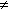Name
• String
• Substring
• Search
Edit
During the searching phase of the Not So Naive algorithm the character comparisons are made with the pattern positions in the following order 1, 2, ... , m-2, m-1, 0.

For each attempt where the window is positioned on the text factor y[j .. j+m-1]: if x=x and xy[j+1] of if xx and x=y[j+1] the pattern is shifted by 2 positions at the end of the attempt and by 1 otherwise.

Thus the preprocessing phase can be done in constant time and space. The searching phase of the Not So Naive algorithm has a quadratic worst case but it is slightly (by coefficient) sub-linear in the average case.

Main features:

• preprocessing phase in constant time and space;

• searching phase in O(nm) time complexity;

• slightly (by coefficient) sub-linear in the average case.

C